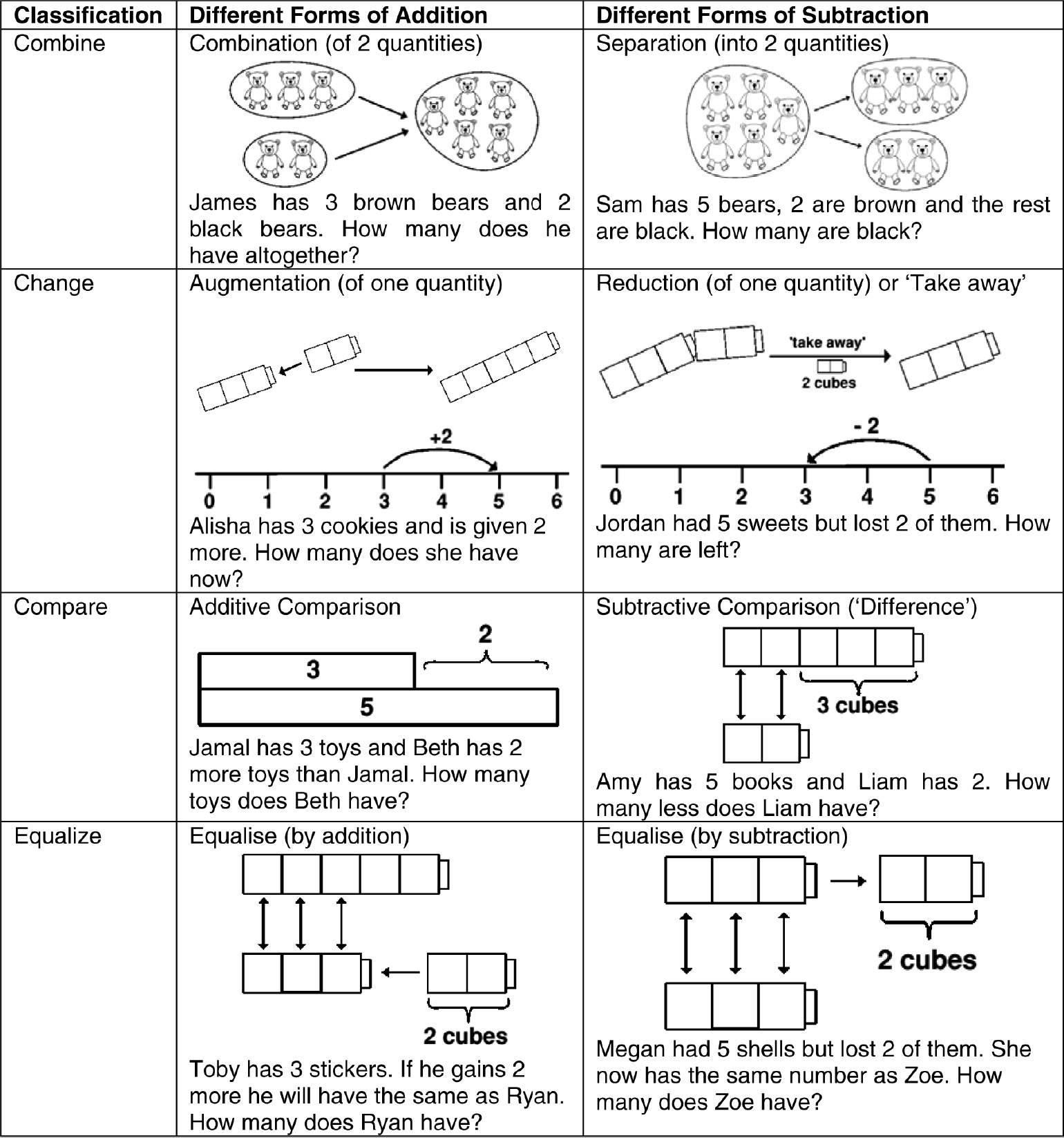‘What a super book! It is absolutely packed with practical ideas and activities to help you love maths, and love teaching and/or learning it. It certainly helps to develop an enthusiasm for a subject most adults tend to say “I'm no good at…”’

# Calculation

Calculation

### Chapter Overview

• Different forms of addition, subtraction, multiplication and division
• How children progress from counting to calculating
• The different mental and written calculation strategies used by children
• Resources and models for supporting understanding of calculation
• The importance of understanding, reliability, efficiency and fluency in calculation.

### Different Forms of Addition and Subtraction

To appreciate the complexities of addition and subtraction we need to recognise that there are different forms of each which children experience. Carpenter and Moser (1983) identified four different types of addition and subtraction contexts: combine, change, compare and equalise (see Figure 10.1). Models and typical word problems are provided to illustrate each form.

### Figure 10.1Forms of Addition and Subtraction (for 3 + 2 = 5 and 5 − 2 = 3)The ...

•••